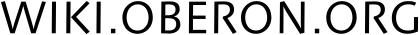#### Инструменты сайта

ob:visual:strings:en

Module for working with strings.

PROCEDURE Length (str: ARRAY OF CHAR): INTEGER;

Returns the number of characters in s up to and excluding the first 0X.

PROCEDURE Insert (source: ARRAY OF CHAR; pos: INTEGER; VAR dest: ARRAY OF CHAR);

Inserts the string 'source' into the string 'dst' at position 'pos' (0 <= pos <= Length(dst)). If pos = Length(dst), 'source' is appended to 'dst'. If the size of 'dst' is not large enough to hold the result of the operation, the result is truncated so that 'dst' is always terminated with 0X.

PROCEDURE Append (str2: ARRAY OF CHAR; VAR str1: ARRAY OF CHAR);

Has the same effect as Insert(s, Length(dst), dst)

PROCEDURE Delete (VAR s: ARRAY OF CHAR; pos, n: INTEGER);

Deletes 'n' characters from 's' starting at position 'pos' (0 <= pos Length(s)). If n > Length(s) - pos, the new length of 's' is 'pos'.

PROCEDURE Replace (source: ARRAY OF CHAR; pos: INTEGER; VAR dest: ARRAY OF CHAR);

Has the same effect as Delete(dst, pos, Length(src)) followed by an Insert(src, pos, dst).

PROCEDURE Extract (source: ARRAY OF CHAR; pos, n: INTEGER; VAR dest: ARRAY OF CHAR);

Extracts a substring 'dst' with 'n' characters from position 'pos' (0 <=pos Length(src)) in 'src'. If n > Length(src) - pos, 'dst' is only the part of 'src' from 'pos' to the end of 'src', i.e. Length(src) -1. If the size of 'dst' is not large enough to hold the result of the operation, the result is truncated so that 'dst' is always terminated with a 0X.

PROCEDURE Pos (pattern, s: ARRAY OF CHAR; pos: INTEGER): INTEGER;

Returns the position of the first occurrence of 'pat' in 's'. Searching starts at position 'pos'. If 'pat' is not found, -1 is returned.

(* conversion functions *)

PROCEDURE IntToString (arg: INTEGER; VAR res: ARRAY OF CHAR);

Represents the integer 'arg' as the string 'res'.

PROCEDURE RealToString (arg: REAL; VAR res: ARRAY OF CHAR);

Represents the real number 'arg' as the string 'res'.

PROCEDURE RealToStringFixed (arg: REAL; VAR res: ARRAY OF CHAR; fixed: INTEGER);

Represents the real number 'arg' as the string 'res' with the number of 'fixed' decimal characters.

PROCEDURE StringToInt (arg: ARRAY OF CHAR; VAR out: INTEGER): BOOLEAN;

Converts a string to an integer.

PROCEDURE StringToReal (arg: ARRAY OF CHAR; VAR out: REAL): BOOLEAN;

Converts a string to a real number.

ob/visual/strings/en.txt · Последнее изменение: 2022/10/02 20:36 — iadenisov

### Инструменты страницы